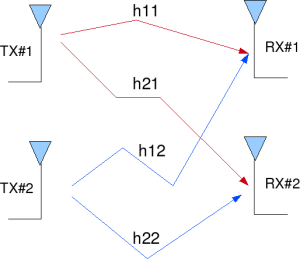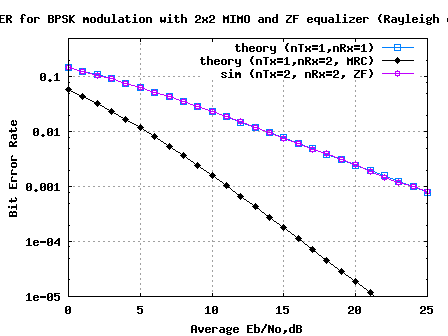- DSP log - http://www.dsplog.com -

MIMO with Zero Forcing equalizer

Posted By Krishna Sankar On October 24, 2008 @ 5:47 am In Channel,MIMO | 154 Comments

We had discussed three Single Input Multiple Output (SIMO also known as receive diversity) schemes – Selection combining , Equal Gain Combining , Maximal Ratio Combining and a Multiple Input Single Output (MISO, also known as transmit diversity) scheme – Alamouti 2×1 STBC.  Let us now discuss the case where there a multiple transmit antennas and multiple receive antennas resulting in the formation of a Multiple Input Multiple Output (MIMO) channel. In this post, we will restrict our discussion to a 2 transmit 2 receive antenna case (resulting in a 2×2 MIMO channel). We will assume that the channel is a flat fading Rayleigh multipath channel  and the modulation is BPSK.

## 2×2 MIMO channel

In a 2×2 MIMO channel, probable usage of the available 2 transmit antennas can be as follows:

1. Consider that we have a transmission sequence, for example

2. In normal transmission, we will be sending in the first time slot, in the second time slot, and so on.

3. However, as we now have 2 transmit antennas, we may group the symbols into groups of two. In the first time slot, send and from the first and second antenna. In second time slot, send and from the first and second antenna, send and in the third time slot and so on.

4. Notice that as we are grouping two symbols and sending them in one time slot, we need only time slots to complete the transmission – data rate is doubled !5. This forms the simple explanation of a probable MIMO transmission scheme with 2 transmit antennas and 2 receive antennas.

Having said this, some of you will wonder – the two transmitted symbols interfered with each other. Can we ever separate the two out? The rest of the post attempts to answer this question.Figure: 2 Transmit 2 Receive (2×2) MIMO channel

## Other Assumptions

1. The channel is flat fading – In simple terms, it means that the multipath channel has only one tap. So, the convolution operation reduces to a simple multiplication. For a more rigorous discussion on flat fading and frequency selective fading, may I urge you to review Chapter 15.3 Signal Time-Spreading from [DIGITAL COMMUNICATIONS: SKLAR]2. The channel experience by each transmit antenna is independent from the channel experienced by other transmit antennas.

3. For the transmit antenna to receive antenna, each transmitted symbol gets multiplied by a randomly varying complex number . As the channel under consideration is a Rayleigh channel, the real and imaginary parts of are Gaussian distributed having mean and variance .

4. The channel experienced between each transmit to the receive antenna is independent and randomly varying in time.

5. On the receive antenna, the noise has the Gaussian probability density function with

with and .

7. The channel is known at the receiver.

## Zero forcing (ZF) equalizer for 2×2 MIMO channel

Let us now try to understand the math for extracting the two symbols which interfered with each other. In the first time slot, the received signal on the first receive antenna is,

.

.

where

, are the received symbol on the first and second antenna respectively,

is the channel from transmit antenna to receive antenna,

is the channel from transmit antenna to receive antenna,

is the channel from transmit antenna to receive antenna,

is the channel from transmit antenna to receive antenna,

, are the transmitted symbols and

is the noise on receive antennas.

We assume that the receiver knows , , and . The receiver also knows and . The unknown s are and . Two equations and two unknowns. Can we solve it? Answer is YES.For convenience, the above equation can be represented in matrix notation as follows:

.

Equivalently,

To solve for , we know that we need to find a matrix which satisfies . The Zero Forcing (ZF) linear detector for meeting this constraint is given by,

.

This matrix is also known as the pseudo inverse  for a general m x n matrix.

The term,

.

## BER with ZF equalizer with 2×2 MIMO

Note that the off diagonal terms in the matrix are not zero (Recall: The off diagonal terms where zero in Alamouti 2×1 STBC  case). Because the off diagonal terms are not zero, the zero forcing equalizer tries to null out the interfering terms when performing the equalization, i.e when solving for the interference from is tried to be nulled and vice versa. While doing so, there can be amplification of noise. Hence Zero Forcing equalizer is not the best possible equalizer to do the job. However, it is simple and reasonably easy to implement.

Further, it can be seen that, following zero forcing equalization, the channel for symbol transmitted from each spatial dimension (space is antenna) is a like a 1×1 Rayleigh fading channel (Refer Section 3.3 of [WIRELESS-TSE, VISWANATH]). Hence the BER for 2×2 MIMO channel in Rayleigh fading with Zero Forcing equalization is same as the BER derived for a 1×1 channel in Rayleigh fading.

For BPSK modulation in Rayleigh fading channel , the bit error rate is derived as,

.

## Simulation Model

The Matlab/Octave script performs the following

(a) Generate random binary sequence of +1′s and -1′s.

(b) Group them into pair of two symbols and send two symbols in one time slot

(c) Multiply the symbols with the channel and then add white Gaussian noise.

(e) Perform hard decision decoding and count the bit errors

(f) Repeat for multiple values of and plot the simulation and theoretical results.Figure: BER plot for 2×2 MIMO channel with ZF equalizer (BPSK modulation in Rayleigh channel)

## Summary

1. As expected, the simulated results with a 2×2 MIMO system using BPSK modulation in Rayleigh channel is showing matching results as obtained in for a 1×1 system for BPSK modulation in Rayleigh channel.

2. As noted in Section 3.3 of [WIRELESS-TSE, VISWANATH], the Zero Forcing equalizer is not the best possible way to equalize the received symbol. The zero forcing equalizer helps us to achieve the data rate gain, but NOT take advantage of diversity gain (as we have two receive antennas).

3. We might not be able to achieve the two fold data rate improvement in all channel conditions. It can so happen that channels are correlated (the coefficients are almost the same). Hence we might not be able to solve for the two unknown transmitted symbols even if we have two received symbols.

4. It is claimed that there can be receiver structures which enables us to have both diversity gain and data rate gain. In future posts, the attempt will be to discuss receiver structures which hopefully enables us to find out approaches which will help us to keep the data rate gain, but still move from the 1×1 curve to 1×2 MRC curve.## References

[DIG-COMM-BARRY-LEE-MESSERSCHMITT] Digital Communication: Third Edition, by John R. Barry, Edward A. Lee, David G. Messerschmitt[WIRELESS-TSE, VISWANATH]Fundamentals of Wireless Communication, David Tse, Pramod ViswanathURL to article: http://www.dsplog.com/2008/10/24/mimo-zero-forcing/

URLs in this post:

 Equal Gain Combining: http://www.dsplog.com/2008/09/19/equal-gain-combining/

 Maximal Ratio Combining : http://www.dsplog.com/2008/09/28/maximal-ratio-combining/

 Alamouti 2×1 STBC.: http://www.dsplog.com/2008/10/16/alamouti-stbc/

 Rayleigh multipath channel: http://www.dsplog.com/2008/07/14/rayleigh-multipath-channel/

 pseudo inverse: http://planetmath.org/encyclopedia/Pseudoinverse.html